# Lua的CPU开销性能优化

## 常见优化

https://www.lua.org/gems/sample.pdf

``````function memoize(f)
local mem = {}
setmetatable(mem, { __mode = "kv" })
return function(x)
local r = mem[x]
if r == nil then
r = f(x)
mem[x] = r
end
return r
end
end
``````

``````local buff = ""
for line in io.lines() do
buff = buff .. line .. "\n"
end

-- 这段代码读取一个350kb大小文件的时候需要约1分钟，同时会移动（开辟后又被回收）50GB内存
``````

``````--如果polyline内有一百万个点，则下面的表结构会占用95kb内存
polyline = {
{x = 1, y = 2},
{x = 3, y = 4},
{x = 5, y = 6},
...
}

--这样优化可以减少到65kb
--只是我们不得不使用polyline来代替比较直观的polyline.x了。
polyline = {
{1, 2},
{3, 4},
{5, 6},
...
}

--这样优化最终可以减少到24kb
--我们必须使用polyline.x来代替polyline.x
polyline = {
x = {1, 3, 5 ... },
y = {2, 4, 6 ... }
}
``````

1）使用闭包代替loadstring()

``````loadstring(string.format("return %d", i))

function oploadstring (k)
return function () return k end
end
``````

loadstring总是会动态地编译传入的字符串，而这个操作是非常耗时的。有时候我们可以使用闭包的形式来达到一样的效果，而且开销更小。

2）使用pairs代替next
next总是从第一个非nil值开始遍历，直到找到一个非nil值，在删除一个表或者一个足够空的表时，很慢。使用next删除一个10万个元素的表时，耗时约为20秒。而使用pairs仅需要0.04秒。

3）不要在for循环中创建表和闭包

4） 重用coroutine

`````` --lua中的coroutine本身是不支持重用机制的，但是我们可以使用一些小技巧来实现它。
co = coroutine.create(function (f)
while f do
f = coroutine.yield(f())
end
end)
``````

5）用C实现一些功能

## 实际案例分析

``````graph = {
[ID]  = { children = { ID1, ID2, ID3 } },
[ID1] = { ... },
[ID2] = { ... },
...
}
``````

``````local origin = 10000
function Util.BFS(fpIdx)
local visited = {}
local Q = {}
local firstNode = BFSNode.new(nil, fpIdx)
Q[#Q + 1] = firstNode
visited[fpIdx] = firstNode
while #Q > 0 do
local node = Q
table.remove(Q, 1)
local v = graph[node.ID]
for i=1, #v.children do
local id = v.children[i]
if id == origin then
local bfsNode = BFSNode.new(node, id)
bfsNode:Log()
return bfsNode:GetPath()
end

if not visited[id] then
local bfsNode = BFSNode.new(node, id)
visited[id] = bfsNode
Q[#Q + 1] = bfsNode
end
end
end
return nil
end
``````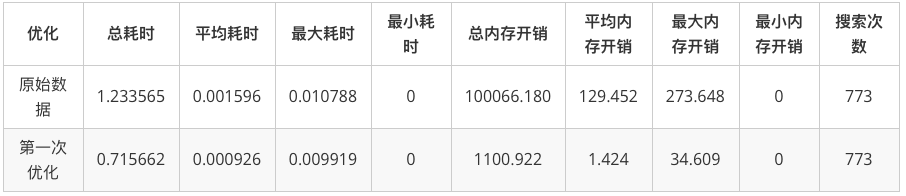``````local origin = 10000
local visited = {}
local queue = {}
local remove = table.removoe
local node
local id = 0
function Util.BFS(fpIdx)
visited = {}
queue = {}
queue[#queue + 1] = fpIdx
visited[fpIdx] = -1
while #queue > 0 do
node = remove(queue, 1)
local v = graph[node]
for i=1, #v.children do
id = v.children[i]
if id == origin then
return Util.GetPath(visited, id, node)
end

if not visited[id] then
visited[id] = node
Q[#Q + 1] = id
end
end
end
return nil
end

function Util.GetPath(visited, targetIdx, parentIdx)
local path = { targetIdx }
while parentIdx > 0 do
path[#path + 1] = parentIdx
parentIdx = visited[parentIdx]
end
return path
end
``````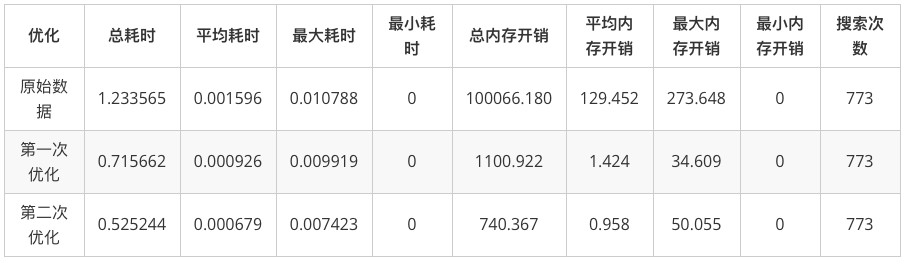``````local noneIdx = 0
local invaildIdx = -1
local origin = 10000
local node
local id = 0
local g = graph
local GetPath = Util.GetPath
local ReuseTable = Util.ReuseTable
local head = 0
local last = 1

function Util.BFS(fpIdx)
head = 0
last = 1
Q[last] = fpIdx
visited[fpIdx] = noneIdx
while last > head do
head = head + 1
node = Q[head]
local v = g[node]
for i=1, #v.children do
id = v.children[i]
if id == origin then
local path = GetPath(visited, id, node)
ReuseTable(visited)
return path
end

if not visited[id] or visited[id] == invaildIdx then
visited[id] = node
last = last + 1
Q[last] = id
end
end
end
ReuseTable(visited)
return nil
end

function Util.GetPath(visited, targetIdx, parentIdx)
local path = { targetIdx }
while parentIdx > 0 do
path[#path + 1] = parentIdx
parentIdx = visited[parentIdx]
end
return path
end

function Util.ReuseTable(t)
for k,_ in pairs(t) do
t[k] = invaildIdx
end
end
``````

``````local pos = obj.transform.position
``````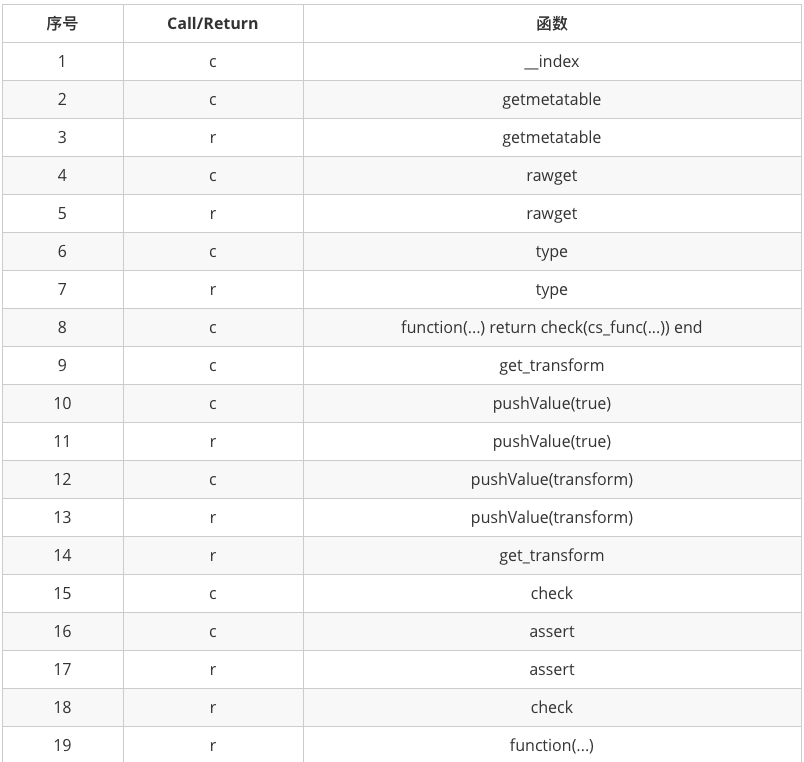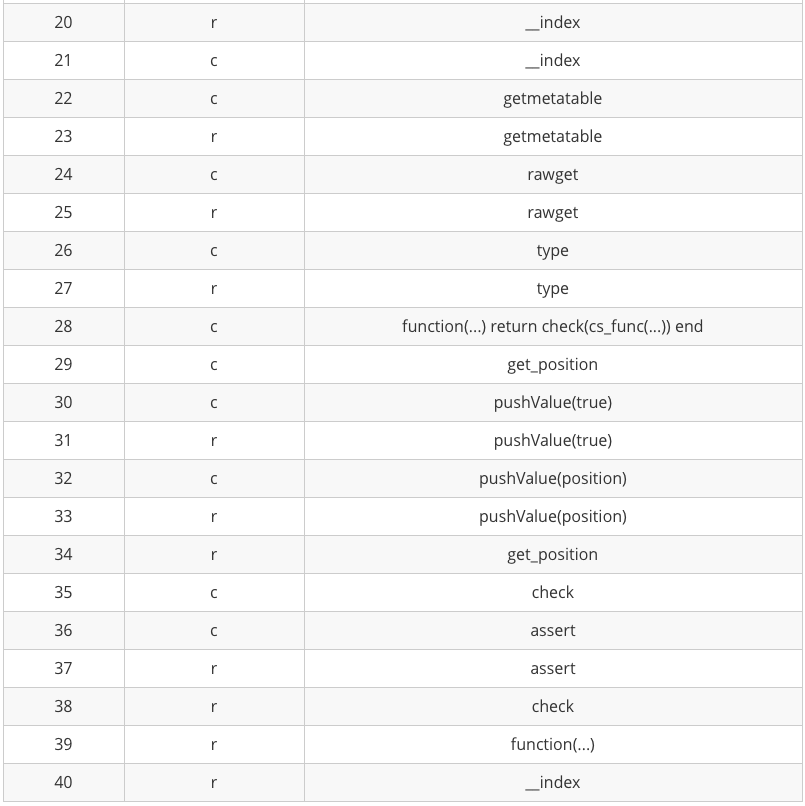1）取得元表中存储的C#函数指针
2）调用C#函数
3）返回结果

``````function Test:Update()
obj.transform.position = LuaVector3.New(0, 0, 0)
end
``````

•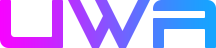UWA2018笔记 | 游戏开发经验分享 发表在 2018年11月07日 回复

[...]http://blog.uwa4d.com/archives/2037.html[...]